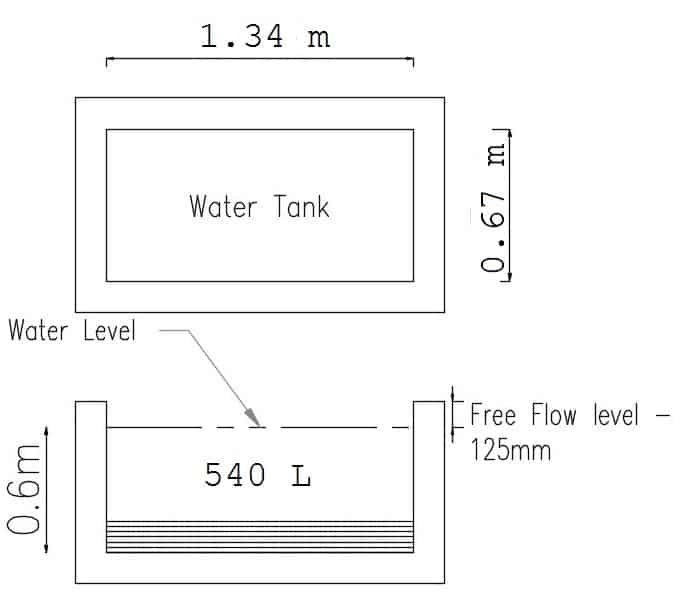# How To Calculate Rectangular Water Tank Size And Capacity

For high rise building, the dimension of water tank along with drawings are provided from architect office.

But for small residential building, we need to calculate by itself. In this article, we will discuss how to calculate rectangular water tank size and capacity.

### Volume of Water Requirement:

As per IS code, a person needs 135 litres water per day for daily use.

Drinking – 5 Litre

Cooking – 5 litres

Bathing and Toilet – 85 litres

Washing clothes and Utensils – 30 litres

House Cleaning – 10 litres

### Calculation Of tank Size and Capacity:

We will calculate for a typical family having 4 members.

Total water requirement = 135 x 4 = 540 litres

To calculate the size of water tank we must know any  one of the following:

Length or width or depth of the tank.

From the formula of volume of water

1 m3 = 1000 litres

1 litre = 0.001 m3

We are requiring 540 litre water

540 litres = 0.54 m3

Let us assume the depth of water tank is 0.6 m.

Area of tank = 0.54/0.6 = 0.9 m2

L x B = 0.9 m2

Now if you know the length or breadth you can put the value on the above formula.

otherwise, take the length as 2 times of B.

L = 2B

2B x B = 0.9 m2

B2 = 0.45

B = 0.67

L = 2 x 0.67 = 1.34 m

So For 540 litre water tank size

Length (L) = 1.34 m

Breatdh (B) = 0.67 m

Depth (D) = 0.6 m